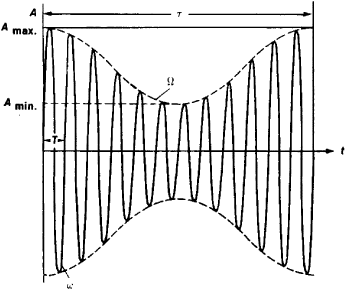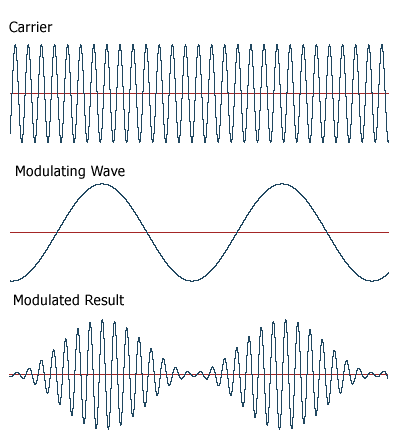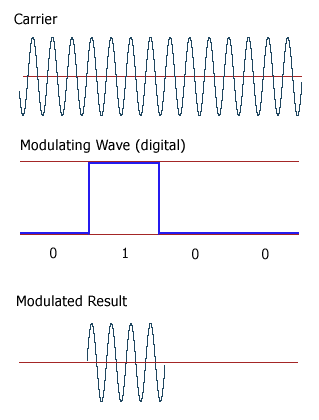# amplitude modulation

(redirected from Amplitude modulated)
Also found in: Dictionary, Thesaurus, Medical.

## amplitude modulation:

see modulationmodulation,
in communications, process in which some characteristic of a wave (the carrier wave) is made to vary in accordance with an information-bearing signal wave (the modulating wave); demodulation is the process by which the original signal is recovered from the wave
transmission or reception of electromagnetic radiation in the radio frequency range. The term is commonly applied also to the equipment used, especially to the radio receiver.
.

## Amplitude Modulation

a variation in the amplitude of oscillations (electrical, mechanical, and others) which takes place at a frequency much lower than the frequency of the oscillations themselves. Amplitude modulation isFigure 1. Harmonic oscillation at a high frequency ω is modulated in amplitude by a harmonic oscillation at a low frequency Ω (τ=1/Ω—itsperiod), t is time, A is the amplitude of the high frequency oscillation, and T is its period.

used in radio technology—for example, in broadcasting. Sound vibrations are converted into electrical oscillations of low frequency Ω (the modulating signal) which periodically vary (modulate) the amplitude of the high frequency oscillations ω (the carrier frequency) generated by a radio transmitter (see Figure 1).

The amplitude-modulated oscillations are radiated in the form of radio waves that are intercepted by radio receivers, where the low frequency modulating oscillations are separated and converted back into an acoustic signal.

## amplitude modulation

[′am·plə‚tüd ‚maj·ə′lā·shən]
(electronics)
Abbreviated AM.
Modulation in which the aplitude of a wave is the characteristic varied in accordance with the intelligence to be transmitted.
In telemetry, those systems of modulation in which each component frequency f of the transmitted intelligence produces a pair of sideband frequencies at carrier frequency plus f and carrier minus f.

## Amplitude Modulation

(communications)
(AM) A method of encoding data by varying the amplitude of a constant frequency carrier.

Contrast Frequency Modulation.

## amplitude modulation

Varying the voltage of a carrier or a direct current in order to transmit analog or digital data. Amplitude modulation (AM) is the oldest method of transmitting human voice electronically. In an analog telephone conversation, the voice waves on both sides are modulating the voltage of the direct current loop connected to them by the telephone company.

Modulate a Carrier
Amplitude modulation (AM) is also widely used to alter a carrier wave to transmit data. For example, in AM radio, the voltage (amplitude) of a carrier with a fixed center frequency (the station's channel) is varied (modulated) by the analog audio signal.

AM is also used for digital data. In quadrature amplitude modulation (QAM), both amplitude and phase modulation are used to create different binary states for transmission (see QAM). AM is also used to modulate light waves in optical fibers. See modulation and carrier.

Vary the AmplitudeIn AM modulation, the voltage (amplitude) of the carrier is varied by the incoming signal. In this example, the modulating wave implies an analog signal.

Digital Amplitude Shift Keying (ASK)For digital signals, amplitude shift keying (ASK) uses two voltage levels for 0 and 1 as in this example.
References in periodicals archive ?
7, PPGP which generates optical amplitude modulated code sequence, preserves the forms of the PPG signals fore and rear fronts.
Since the test requires that the RF test field is sine wave amplitude modulated, the input level is effectively increasing and decreasing around the nominal test level.
Phase and/or amplitude modulated signals are often used for information transmission in microwave communications systems.
Idea is to use one optical source and external modulator that is driven by amplitude modulated RF signal.
The first type consists of an attenuator in cascade with a 360 [degrees] phase shifter. The second type is a tandem modulator consisting of two orthogonal channels that are individually amplitude modulated and then power combined in phase.
Since the network analyzer output is amplitude modulated, many test devices will generate spurious products that are also amplitude modulated.
Measurement of amplitude modulated (AM) and phase modulated (PM) noise signature has always been a troublesome procedure for engineers.
The input pulse will actually be a sine wave RF signal that is amplitude modulated. The RF pulse is then the product of the modulation term f(t) and a cosine term that is a function of the RF carrier frequency |f.sub.RF~, time t and an arbitrary phase angle |alpha~.
Figure 1a shows the spectrum of an amplitude modulated signal and a direct sequence spread spectrum signal having the same carrier frequency of 30 MHz.

Site: Follow: Share:
Open / Close